Chapter Notes: Light – Reflection & Refraction

# Light – Reflection & Refraction Chapter Notes - Science Class 10

 Table of contentsReflection of LightSpherical MirrorsRefraction of LightLaws of Refraction of LightWhat is Refractive Index?LensesImage Formation for Convex LensImage Formation for Concave LensLens FormulaPower of LensMagnification of LensImagine you're holding a flashlight in a dark room. When you turn it on, you see a bright beam of light. Well, light is a form of energy that makes things visible to our eyes. It's like a messenger that carries information about the world around us.
Light is a form of electromagnetic energy that causes the sensation of vision. Some common phenomena associated with lights are image formation by mirrors, the twinkling of stars, the beautiful colours of a rainbow, bending of light by a medium and many more.

Properties of Light

• Electromagnetic wave, so does not require any medium to travel.
• Light tends to travel in straight line.
• Light has dual nature i.e. wave as well as particle.
• Speed of light is maximum in vaccum. Its value is 3 × 108 ms-1.
• When light falls on a surface, following may happen:
• Reflection
• Refraction
• Absorption

## Reflection of Light

Have you ever wondered why you can see your reflection in a shiny surface? The answer lies in the phenomenon of reflection. When light encounters a surface, it bounces back, allowing us to see objects and ourselves.

The reflection of light is the phenomenon where light bounces off a surface and changes direction.

Laws of Reflection
(i) Angle of incidence is equal to the angle of reflection.
(ii) The incident ray, the reflected ray and the normal at the point of incidence, all lie in the same plane.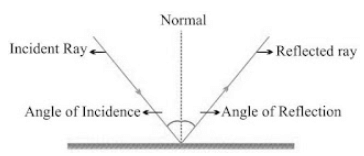Virtual and Real image

Image is a point where at least two light rays actually meet or appear to meet.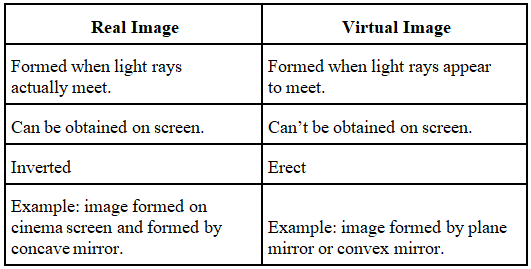Question for Chapter Notes: Light – Reflection & Refraction
Try yourself:
Which phenomenon is responsible for allowing us to see our reflection in a shiny surface?

### Image Formed by Plane Mirror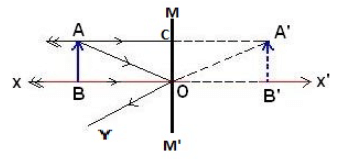Characteristics of Image formed by Plane Mirror
(i)  Virtual and erect.
(ii) Size of image is equal to the size of object.
(iii) Image is formed as far behind the mirror as the object is in front of it.
(iv) Laterally inverted.

Lateral Inversion: The right side of the object appears left side of the image and vice-versa.

Application of lateral inversion

• The word AMBULANCE is written in reverse direction so that it can be read correctly in rear view mirror of vehicles going in front of it.## Spherical Mirrors

• Mirrors whose reflecting surface is curved.
• There are two types of spherical mirrors:
(i) Convex Mirror
(ii) Concave Mirror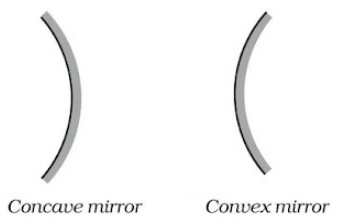Properties of Concave mirror

• Reflecting surface is curved inwards.
• Converging mirror

Properties of Convex mirror

• Reflecting surface is curved outwards.
• Diverging mirror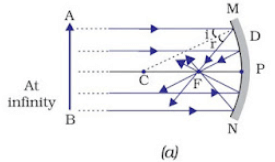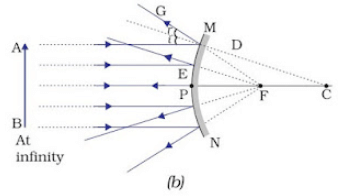Common terms for Spherical mirrors

• Principal axis: The line joining the pole and center of curvature.
• Pole (P): The centre of the spherical mirror.
• Aperture (MN):  It is the effective diameter of the spherical mirror.
• Center of Curvature (C): The centre of the hollow glass sphere of which the mirror was a part.
• Radius of Curvature (R): The distance between the pole and the centre of curvature.
• Focus (F): The point on principal axis where all the parallel light rays actually meet or appear to meet after reflection.
• Focal length (f): The distance between the pole and the focus.
• Relationship between focal length and radius of curvature: f = R/2

### Rules for making ray diagrams by spherical mirror

(i)  A ray parallel to the principal axis, after reflection, will pass through the principal focus in case of a concave mirror or appear to diverge from the principal focus in case of a convex mirror.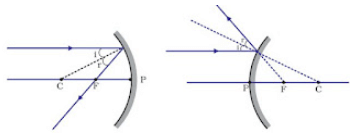(ii) A ray passing through the principal focus of a concave mirror or a ray which is directed towards the principal focus of a convex mirror, after reflection, will emerge parallel to the principal axis.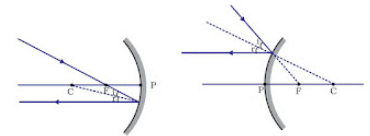(iii) A ray passing through the centre of curvature of a concave mirror or directed in the direction of the centre of curvature of a convex mirror, after reflection, is reflected back along the same path.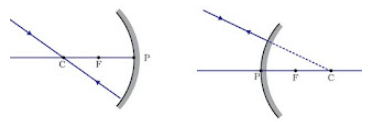(iv) A ray incident obliquely to the principal axis, towards a point P (pole of the mirror), on the concave mirror or a convex mirror, is reflected obliquely. The incident and reflected rays follow the laws of reflection at the point of incidence (point P), making equal angles with the principal axis.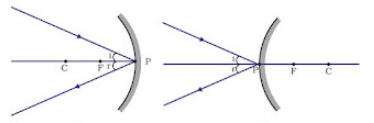Question for Chapter Notes: Light – Reflection & Refraction
Try yourself:
Which type of mirror has a reflecting surface curved inwards?

### Ray diagrams for images formed by concave mirror

(i) When object is at infinity
Image Position − At ‘F’
Nature of image – Real, inverted
Size – Point sized or highly diminished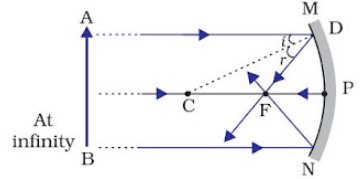(ii) When object is beyond ‘C’
Image Position – Between ‘F’ and ‘C’
Nature of image – Real, inverted
Size – Diminished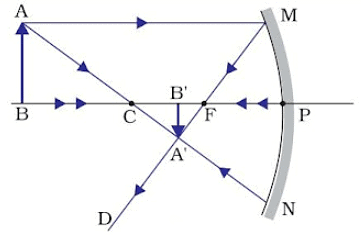(iii) When object is at ‘C’
Image Position – At ‘C’
Nature of image – Real, inverted
Size – Same size as that of object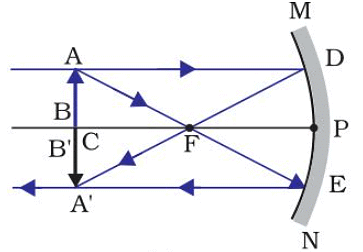(iv) When object is placed between ‘F’ and ‘C’
Image Position – Beyond ‘C’
Nature of image– Real, inverted
Size – Enlarged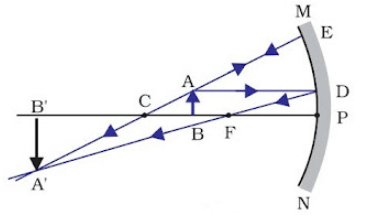(v) When object is placed at ‘F’
Image Position – At Infinity
Nature of image – Real, inverted
Size – Highly enlarged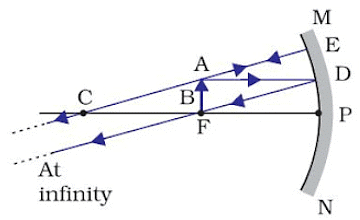(vi) When object is between ‘P’ and ‘F’
Image Position – Behind the mirror
Nature of image – Virtual, erect
Size – Enlarged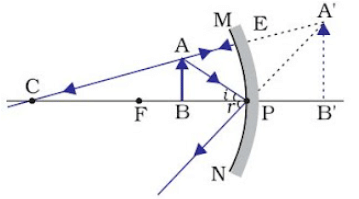### Uses of Concave Mirror

• Used in torches, search lights and vehicles headlights to get powerful parallel beam of light.
• Concave mirrors are used by dentists to see large image of teeth of patients. (Teeth have to be placed between pole and focus).
• Concave mirror is used as shaving mirror to see a larger image of the face.
• Large concave mirrors are used to concentrate sunlight to produce heat in solar furnace.

### Ray diagrams of images formed by convex mirror

(i)  When object is placed at infinity
Image Position − At ‘F’
Nature of image – Virtual, erect
Size – Point sized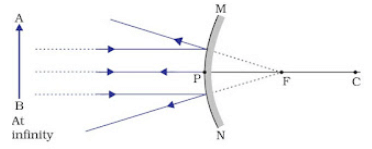(ii) When object is placed between pole and infinity
Image Position – Between ‘P’ and ‘F’
Nature of image– Virtual, erect
Size – Diminished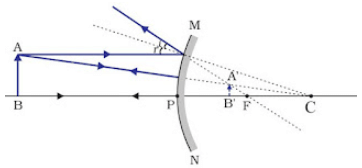A full length image of a tall building/tree can be seen in a small convex mirror.

Uses of Convex Mirror

• Convex mirrors are used as rear view mirrors in vehicles because
• they always give an erect though diminished image.
• they have a wider field of view as they are curved outwards.
• Convex mirrors are used at blind turns and on points of merging traffic to facilitate vision of both side traffic.
• Used in shops as security mirror.

### Sign Convention for Reflection by Spherical Mirror

• The object is placed to the left of the mirror.
• All distances parallel to the principal axis are measured from the pole of the mirror.
• All distances measured in the direction of incident ray (along + X-axis) are taken as positive and those measured against the direction of incident ray (along – X-axis) are taken as negative.
• Distance measured perpendicular to and above the principal axis are taken as positive.
• Distances measured perpendicular to and below the principal axis are taken as negative.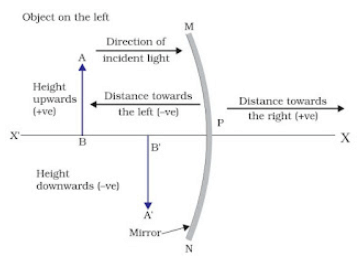• Object distance = ‘u’ is always negative.
• Focal length of concave mirror = Negative
• Focal length of convex mirror = Positive

### Mirror Formula

1/v + 1/u = 1/f
where, v = Image distance
u = Object distance
f = Focal length

Magnification of Spherical Mirrors

It is the ratio of the height of image to the height of object.
m = Height of image/Height of object
⇒ m = hi/ho
Also, m = -v/u

• If ‘m’ is negative, image is real.
• If ‘m’ is positive, image is virtual.
• If hi = ho then m = 1, i.e., image is equal to object.
• If hi > ho then m > 1 i.e., image is enlarged.
• If hi < ho then m < 1 i.e., image is diminished.

Magnification of plane mirror is always + 1.
‘1’ indicates that image is equal to object’s size.

• If ‘m’ is ‘+ve’ and less than 1, it is a convex mirror.
• If ‘m’ is ‘+ve’ and more than 1, it is a concave mirror.
• If ‘m’ is ‘-ve’, it is a concave mirror.

## Refraction of Light

The bending of a ray of light as it passes from one medium to another is called refraction.

It is due to a change in the velocity of light while travelling from one medium to another.

• The maximum velocity of light is 3 x 108 m/sec in a vacuum or air.
• The velocity is less in the denser medium.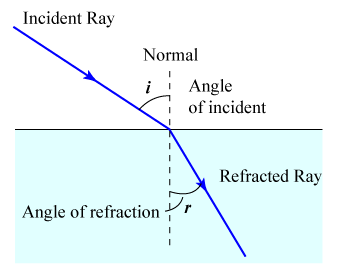## Laws of Refraction of Light

Laws of refraction state that:

• The incident ray refracted ray, and the normal to the interface of two media at the point of incidence all lie on the same plane.
• The ratio of the sine of the angle of incidence to the sine of the angle of refraction is constant. This is also known as Snell’s law of refraction.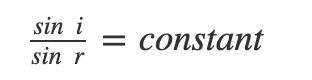### Bending of Light Ray

According to Snell's law, μ sin i = μsin r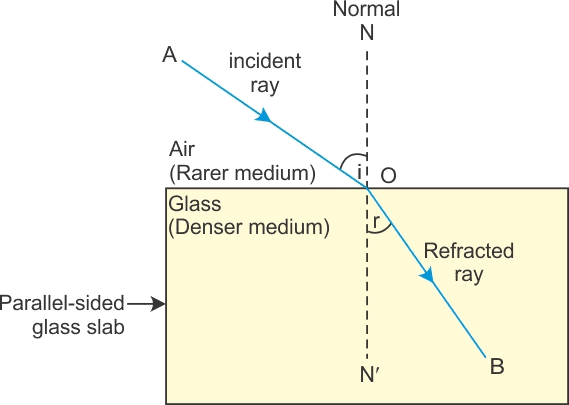(i) If light passes from rarer to the denser medium:
μ = μ and μ= μ
so that,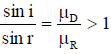⇒ ∠i > ∠r
In passing from rarer to a denser medium, the ray bends towards the normal.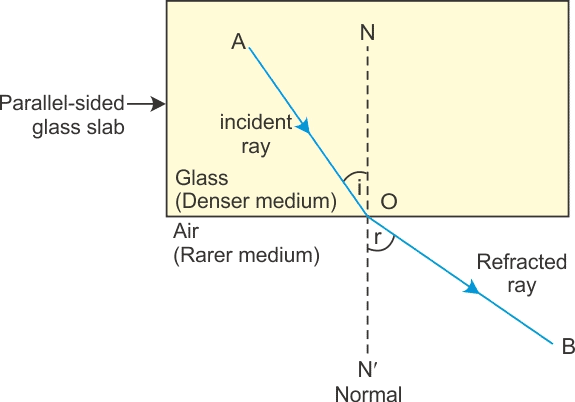(ii) If light passes from denser to rarer medium μ1= μand μ= μ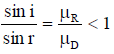⇒ ∠ i < ∠r
In passing from denser to rarer medium, the ray bends away from the normal.
Refractive index depends on nature and density of medium and colour of light refractive index is maximum for violet and minimum for the red light.

## What is Refractive Index?

Refractive index, also called the index of refraction describes how fast light travels through the material.

Refractive Index is dimensionless. For a given material, the refractive index is the ratio between the speed of light in a vacuum (c) and the speed of light in the medium (v). If the refractive index for a medium is represented by n, then it is given by the following formula: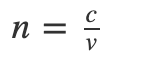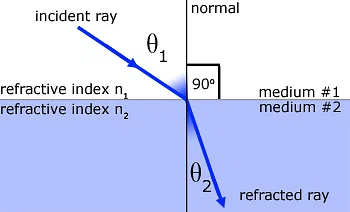• When a light ray is refracted from medium number 1 into medium number 2, the relative refractive index of medium 2 w.r.t. medium 1(n21) is given by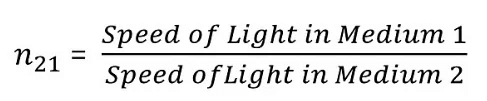• Similarly, the refractive index of medium 1 with respect to medium 2 is given by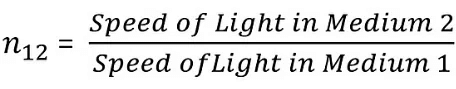• Therefore, we conclude that n21 x n12 = 1
or  n21 = 1 / n12

## Lenses

• A lens is a piece of any transparent material bounded by two curved surfaces or by one curved and one plane surface.
• Lens are of two types:
Convex or convergent lens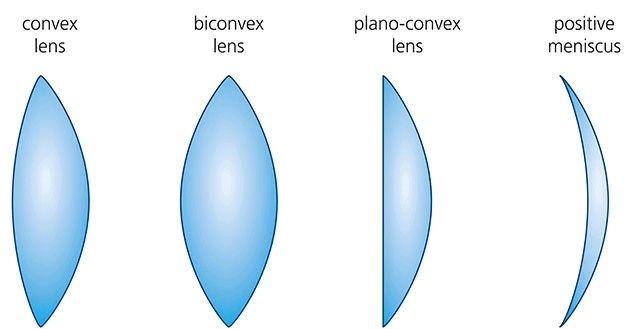Concave or divergent lens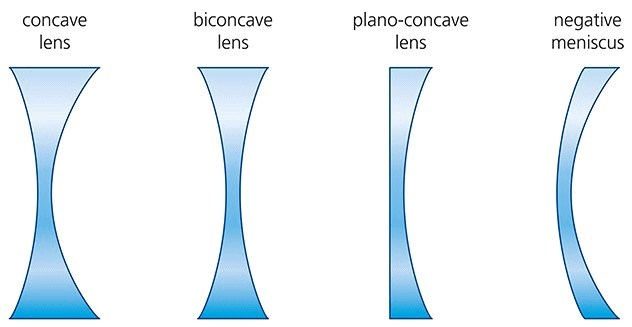### Terminologies

• Optical Centre: O is a point for a given lens through which any ray passes undeviated.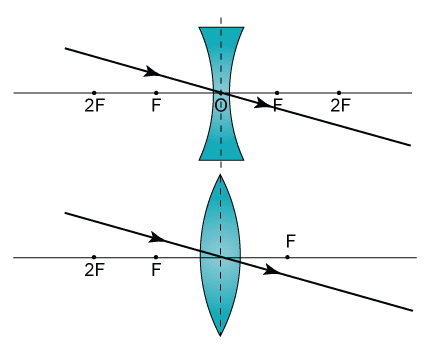• Principal Axis: C1C2  is a line passing through optical centre and perpendicular to the lens.
• Principal Focus: A lens has two surfaces and hence two focal points. First focal point is an object point on the principal axis for which image is formed at infinity.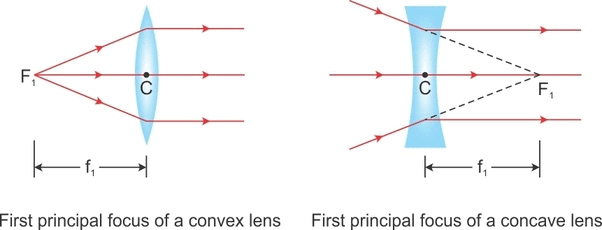• While second focal point is an image point on the principal axis for which object lies at infinity.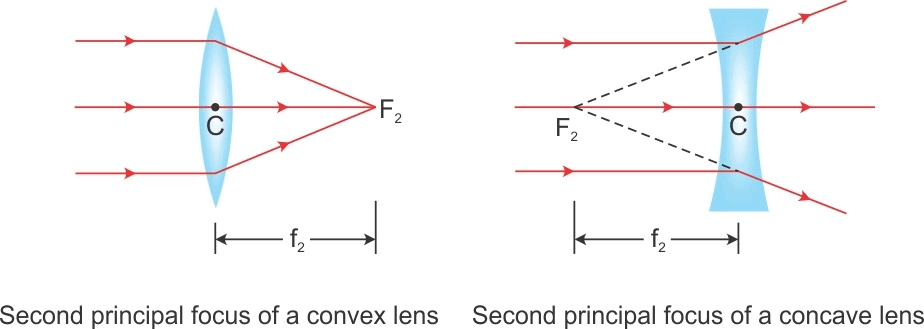• Focal Length f is defined as the distance between optical centre of a lens and the point where the parallel beam of light converges or appears to converge.
• Aperture : In reference to a lens, aperture means the effective diameter. Intensity of image formed by a lens which depends on the light passing through the lens will depend on the square of aperture, i.e., I µ (Aperture)

[Intext Question]

### Rules for Image Formation

• A ray passing through optical centre proceeds undeviated through the lens.
• A ray passing through first focus or directed towards it, after refraction from the lens, becomes parallel to the principal axis.
• A ray passing parallel to the principal axis after refraction through the lenses passes or appears to pass through F2.

For Convergent or Convex Lens:

 Object Image Magnification ∞ F m << – 1 ∞ – 2F F – 2F m < –1 2F 2F m = –1 F – 2F ∞ – 2F m > –1 F ∞ m >> –1 F – O In front of lens m > + 1

## Image Formation for Convex Lens

• Object is placed at infinity.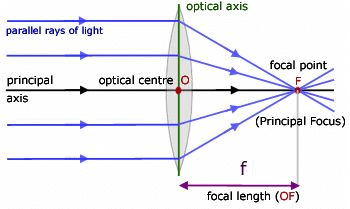Image: at F real, inverted, very small in size and m << - 1
• Object is placed in between ∞ -2F.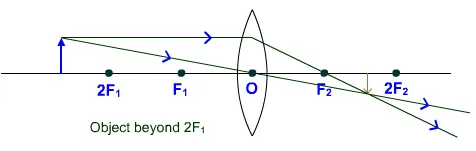Image: real (F - 2F) inverted, small in size (diminished), and m < - 1
• Object is placed at 2F.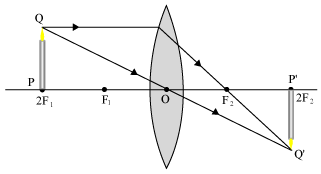Image : real (at 2F)
inverted
equal (of same size)
(m = -1)
• Object is placed in between 2F - F.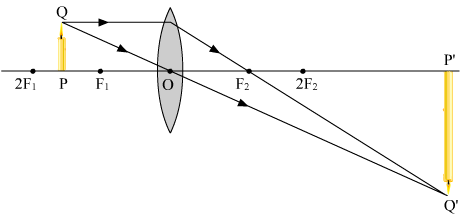Image : real (2F - ∞)
inverted
enlarged
m > 1
• Object is placed at F.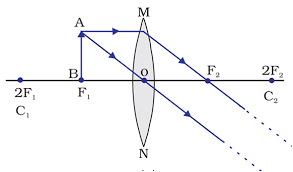• Object is placed in between F - O.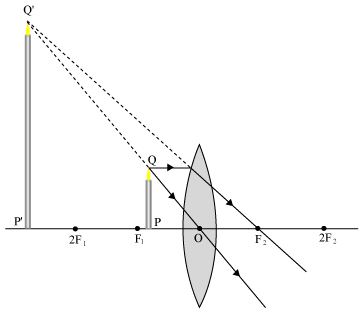Image: virtual (in front of lens)
erected
enlarge
(m > + 1)
 Given Real Virtual u - - v + - h1 + + h2 - + m - +

## Image Formation for Concave Lens

Image is virtual, diminished, erect, towards the object, m = +ve

• Object is placed at infinity.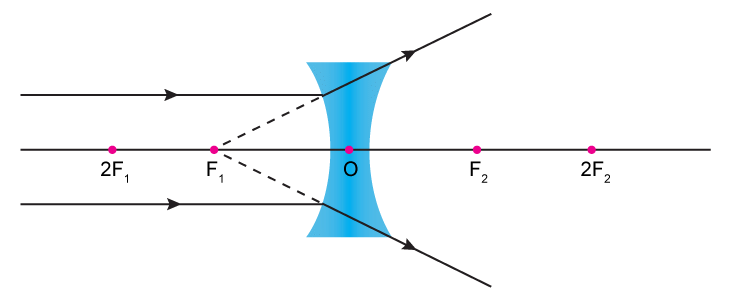Image: At F
virtual
erected
diminished
(m << + 1)
• Object is placed in front of the lens.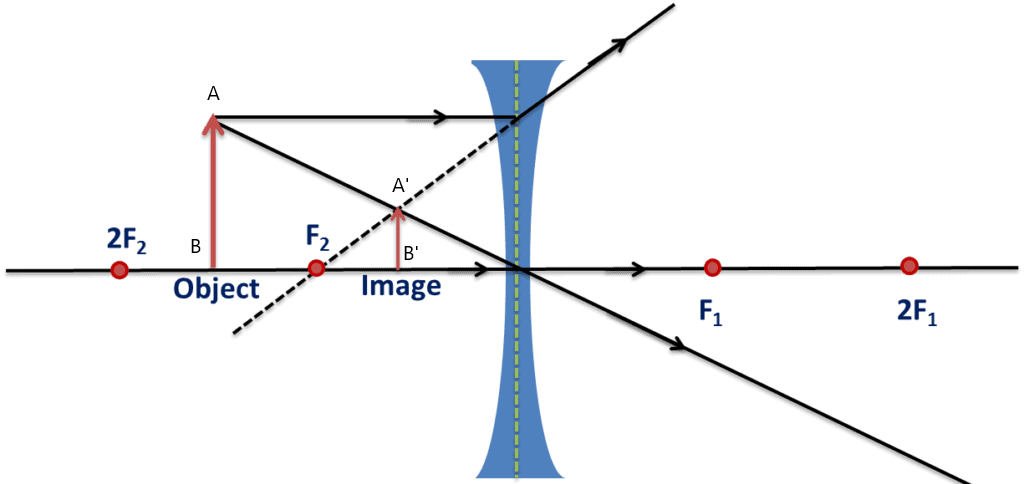Image: between F and optical centre
virtual
erected
diminished
(m < + 1)

## Lens Formula

Focal length of a lens can be found by the following formula: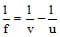Where - f = Focal length of lens.

v = Distance of image from pole

u = Distance of object from pole.

### Uses of Lens Formula

(i) Put the correct signs of known variables according to the sign conventions.

(ii) Do not put the sign of an unknown variable. The sign will automatically show up during calculations.

(iii) If the calculated sign of a variable turns out positive, then the variable calculated is on the other side of the lens, i.e., on the opposite side to the object. However, if the calculated variable is of negative sign, then it is on the same side as the object.

### Combination of lenses

Two thin lens are placed in contact to each other

Power of combination: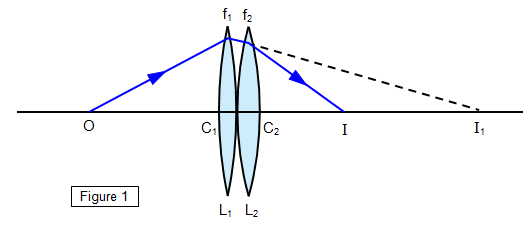P = P1 + P2

1/F =  1/f+ 1/f2

Use sign convention when solving numericals.

Two thin lenses are placed at a small distance a.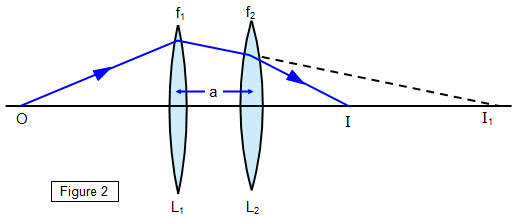1/F = 1/f+ 1/f2 - a/f1f2

P = P1 + P2 - a P1P2

Use sign convention when solving numericals.

## Power of Lens

The power of a lens is defined as the reciprocal of focal length of the lens. Focal length should always be measured in meters.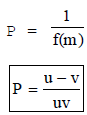Unit of power of lens is 1/meter which is called dioptre.

## Magnification of Lens

The magnification is defined as the ratio of the height of the image and the height of the object it is represented by M.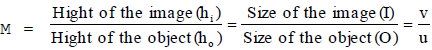The document Light – Reflection & Refraction Chapter Notes | Science Class 10 is a part of the Class 10 Course Science Class 10.
All you need of Class 10 at this link: Class 10

## Science Class 10

78 videos|509 docs|153 tests

## FAQs on Light – Reflection & Refraction Chapter Notes - Science Class 10

 1. What is the difference between reflection and refraction of light?Ans. Reflection of light refers to the bouncing back of light rays from a surface, whereas refraction of light is the bending of light rays when they pass from one medium to another.
 2. How does a convex lens form an image?Ans. A convex lens is thicker in the middle and thinner at the edges. When parallel rays of light pass through a convex lens, they converge and meet at a point called the focal point. This convergence of light rays forms a real and inverted image.
 3. What is the refractive index of a medium?Ans. Refractive index is a measure of how much light bends when it passes from one medium to another. It is the ratio of the speed of light in a vacuum to the speed of light in the medium. It is denoted by the symbol "n" and has no unit.
 4. How is the image formed by a concave lens different from that formed by a convex lens?Ans. A concave lens is thinner in the middle and thicker at the edges. When parallel rays of light pass through a concave lens, they diverge and do not meet. This divergence of light rays forms a virtual and upright image, which is smaller in size compared to the object.
 5. What is the lens formula?Ans. The lens formula gives the relationship between the object distance (u), image distance (v), and focal length (f) of a lens. It is given by 1/f = 1/v - 1/u. This formula helps in calculating the position, size, and nature of the image formed by a lens.

## Science Class 10

78 videos|509 docs|153 testsExplore Courses for Class 10 examSignup to see your scores go up within 7 days! Learn & Practice with 1000+ FREE Notes, Videos & Tests.
10M+ students study on EduRev
Track your progress, build streaks, highlight & save important lessons and more!
Related Searches

,

,

,

,

,

,

,

,

,

,

,

,

,

,

,

,

,

,

,

,

,

;